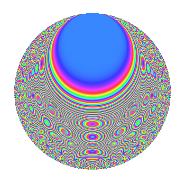# Properties

 Label 1350.2.mLevel 1350 Weight 2 Character orbit m Rep. character $$\chi_{1350}(109,\cdot)$$ Character field $$\Q(\zeta_{10})$$ Dimension 160 Sturm bound 540

# Related objects

## Defining parameters

 Level: $$N$$ = $$1350 = 2 \cdot 3^{3} \cdot 5^{2}$$ Weight: $$k$$ = $$2$$ Character orbit: $$[\chi]$$ = 1350.m (of order $$10$$ and degree $$4$$) Character conductor: $$\operatorname{cond}(\chi)$$ = $$25$$ Character field: $$\Q(\zeta_{10})$$ Sturm bound: $$540$$

## Dimensions

The following table gives the dimensions of various subspaces of $$M_{2}(1350, [\chi])$$.

Total New Old
Modular forms 1128 160 968
Cusp forms 1032 160 872
Eisenstein series 96 0 96

## Trace form

 $$160q + 40q^{4} + O(q^{10})$$ $$160q + 40q^{4} - 10q^{10} - 40q^{16} + 12q^{19} + 20q^{22} - 26q^{25} + 10q^{28} - 6q^{31} - 24q^{34} + 40q^{37} + 10q^{40} - 12q^{46} - 100q^{49} - 58q^{55} - 40q^{61} + 40q^{64} + 40q^{67} + 98q^{70} + 8q^{76} + 56q^{79} + 144q^{85} - 10q^{88} - 20q^{91} - 16q^{94} + 10q^{97} + O(q^{100})$$

## Decomposition of $$S_{2}^{\mathrm{new}}(1350, [\chi])$$ into newform subspaces

The newforms in this space have not yet been added to the LMFDB.

## Decomposition of $$S_{2}^{\mathrm{old}}(1350, [\chi])$$ into lower level spaces

$$S_{2}^{\mathrm{old}}(1350, [\chi]) \cong$$ $$S_{2}^{\mathrm{new}}(25, [\chi])$$$$^{\oplus 8}$$$$\oplus$$$$S_{2}^{\mathrm{new}}(50, [\chi])$$$$^{\oplus 4}$$$$\oplus$$$$S_{2}^{\mathrm{new}}(75, [\chi])$$$$^{\oplus 6}$$$$\oplus$$$$S_{2}^{\mathrm{new}}(150, [\chi])$$$$^{\oplus 3}$$$$\oplus$$$$S_{2}^{\mathrm{new}}(225, [\chi])$$$$^{\oplus 4}$$$$\oplus$$$$S_{2}^{\mathrm{new}}(450, [\chi])$$$$^{\oplus 2}$$$$\oplus$$$$S_{2}^{\mathrm{new}}(675, [\chi])$$$$^{\oplus 2}$$

## Hecke Characteristic Polynomials

There are no characteristic polynomials of Hecke operators in the database